Download the eSaral app and start learning from Kota's top IITians and doctors.

# NCERT Solutions for Class 9 Maths chapter 14 Exercise 14.4 - Statistics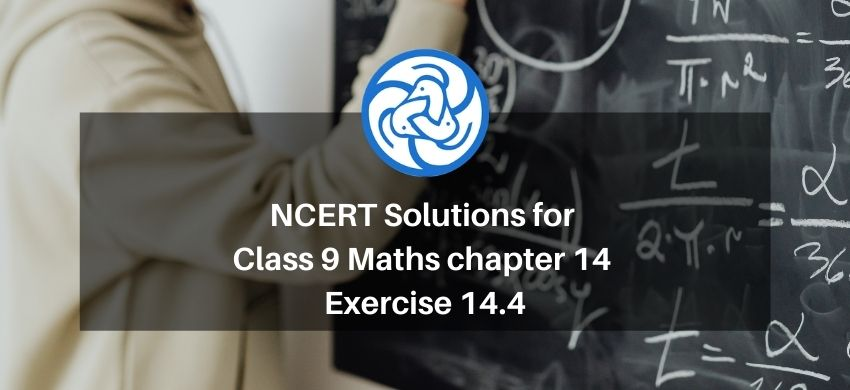Hey, are you a class 9 Student and Looking for Ways to Download NCERT Solutions for Class 9 Maths chapter 14 Exercise 14.4? If Yes then you are at the right place.

Here we have listed Class 9 maths chapter 14 exercise 14.4 solutions in PDF that is prepared by Kota’s top IITian’s Faculties by keeping Simplicity in mind.

If you want to score high in your class 9 Maths Exam then it is very important for you to have a good knowledge of all the important topics, so to learn and practice those topics you can use eSaral NCERT Solutions.

So, without wasting more time Let’s start.

### Download The PDF of NCERT Solutions for Class 9 Maths chapter 14 Exercise 14.4 "Statistics"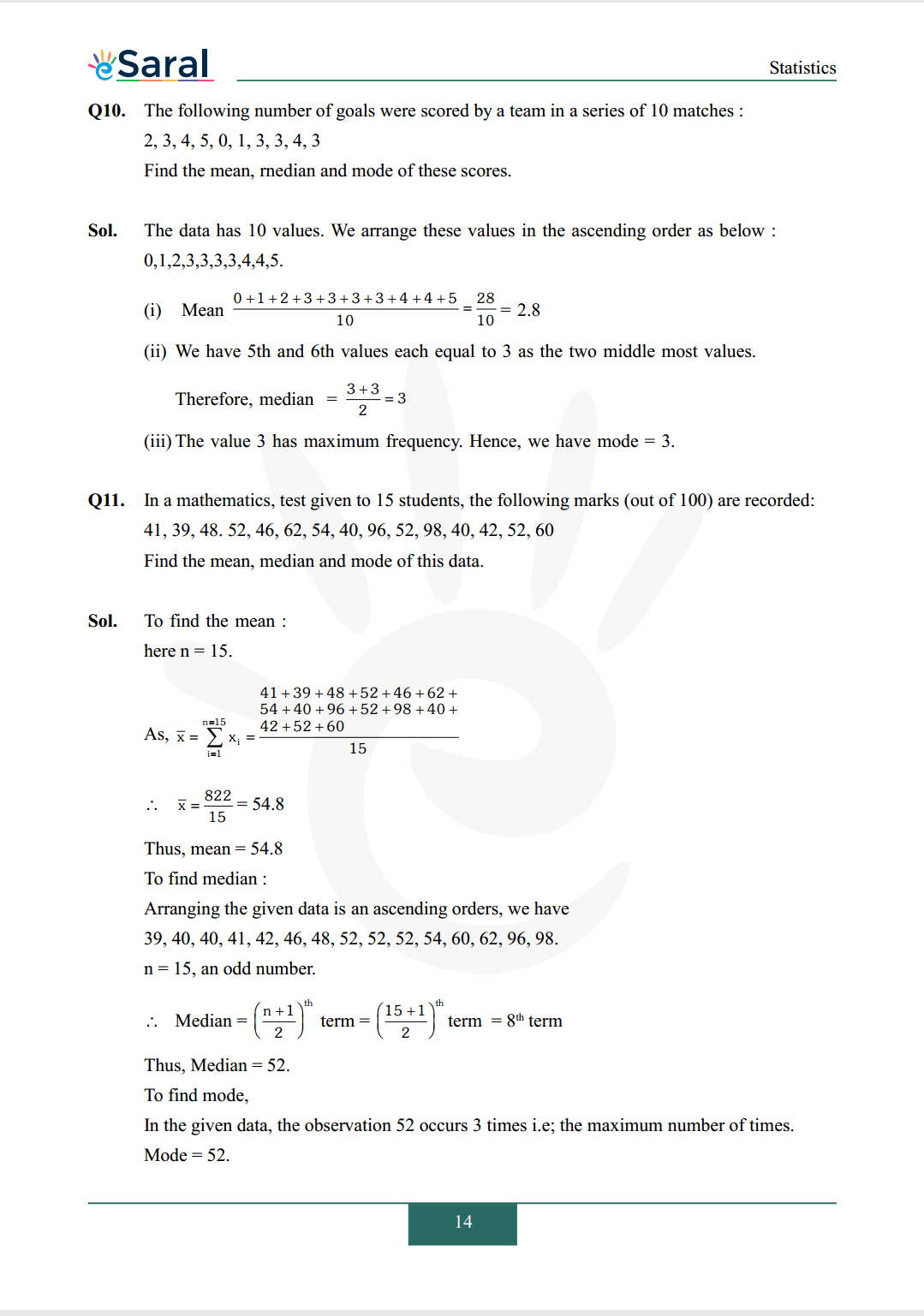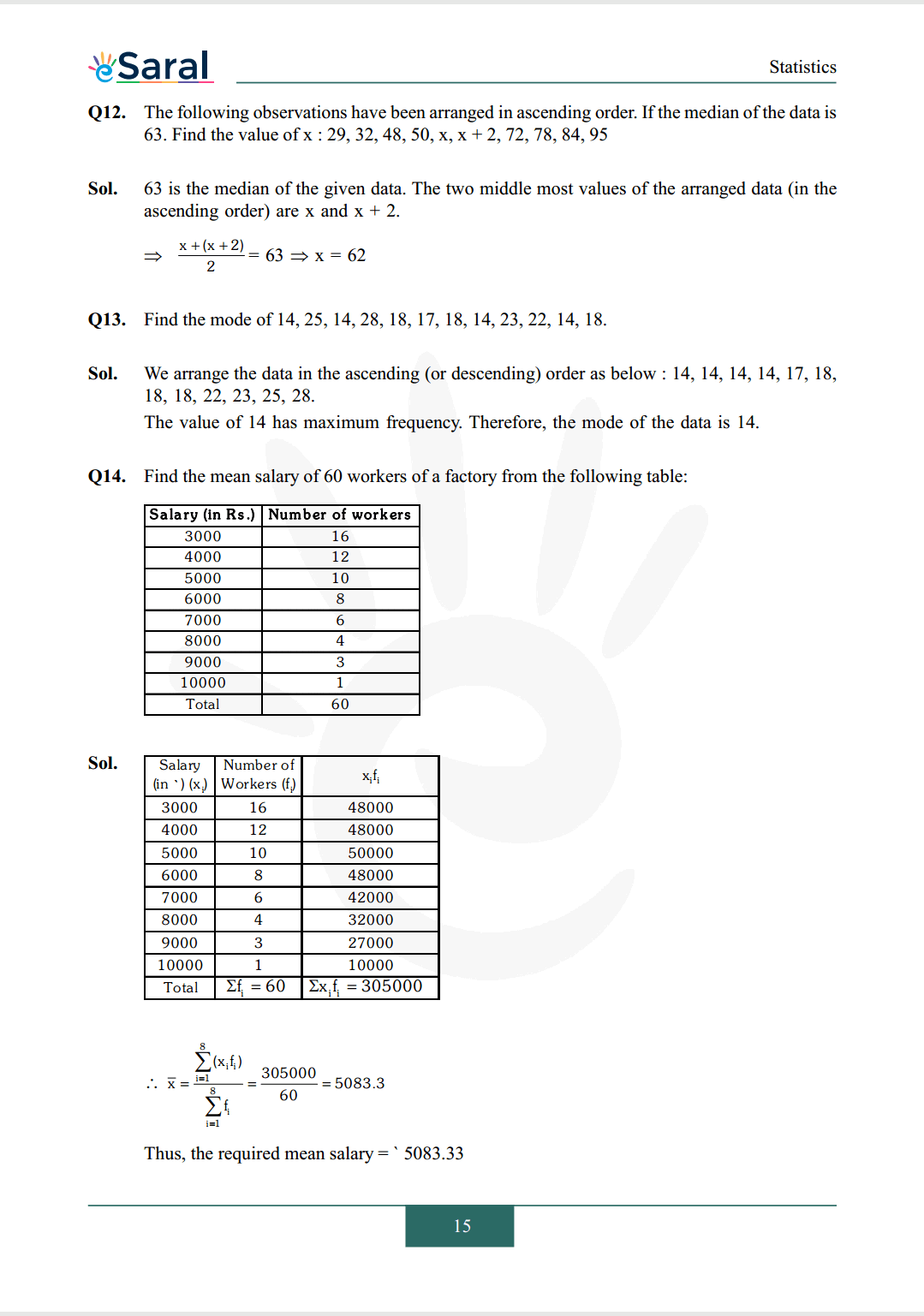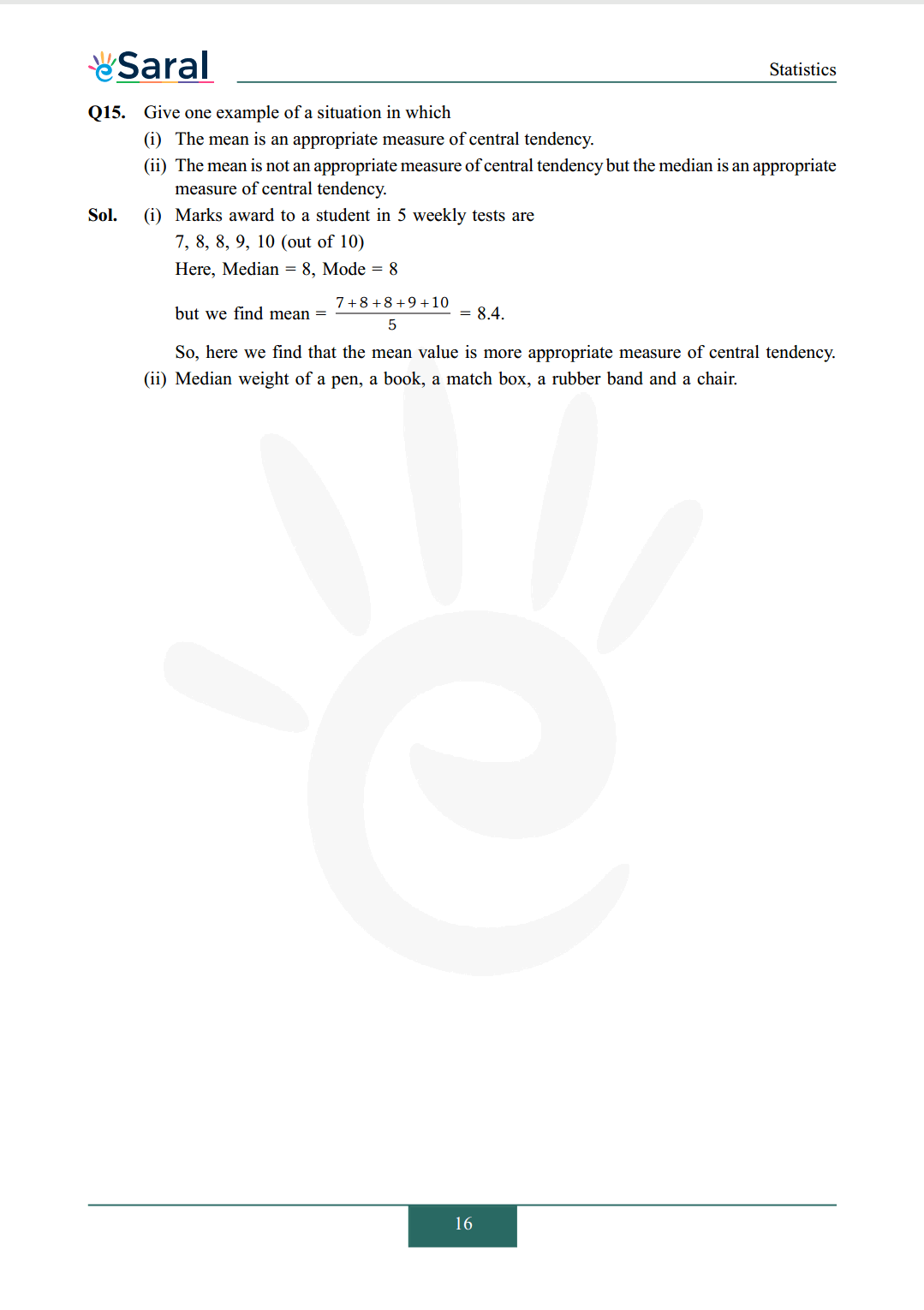#### All Questions of Chapter 14 Exercise 14.4

Once you complete the chapter 14 then you can revise Ex. 14.4 by solving following questions

Q1. The following number of goals were scored by a team in a series of 10 matches : 2, 3, 4, 5, 0, 1, 3, 3, 4, 3
Find the mean, rnedian and mode of these scores.

Q2. In a mathematics, test given to 15 students, the following marks (out of 100) are recorded: 41, 39, 48. 52, 46, 62, 54, 40, 96, 52, 98, 40, 42, 52, 60
Find the mean, median and mode of this data.

Q3. The following observations have been arranged in ascending order. If the median of the data is
63. Find the value of x : 29, 32, 48, 50, x, x + 2, 72, 78, 84, 95

Q4. Find the mode of 14, 25, 14, 28, 18, 17, 18, 14, 23, 22, 14, 18.

Q5. Find the mean salary of 60 workers of a factory from the following table: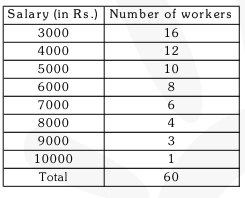Q6. Give one example of a situation in which
(I) The mean is an appropriate measure of central tendency.
(ii) The mean is not an appropriate measure of central tendency but the median is an appropriate measure of central tendency.